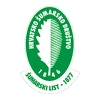DIGITALNA ARHIVA ŠUMARSKOG LISTA
prilagođeno pretraživanje po punom tekstu

 ŠUMARSKI LIST 11-12/2014 str. 34     <-- 34 -->        PDF 3. Results Rezultati 3.1 Exploratory data analysis – Preliminarna analiza podataka Descriptive statistics of the sampled trees, for each site type and for the study area as a whole, are given in Table 3. Mean volume was statistically significantly different between site types, (F = 28.214, p = 0.000), justifying the development of volume estimation models for each site type separately. Summary statistics for the nearest neighbors to the sampled trees (distances and species) are given to tables 4 and 5, respectively. 3.2 Selection of the best regression model and validation for site type A – Odabir najpovoljnijeg modela regresije za lokaciju A Based on the results of Table 6, for site type A, model 2 should be rejected, because, although is adequately fitted, has negative R2 for validation data. Also, models 4 and 5 should be rejected, because, although they have fairly good values for comparison criteria, both for fitting and validation data, some of their regression coefficients are not statistically significant at the level p<0.05 (Table 7). Moreover, Model 1 is inappropriate, because regression coefficients are not statistically significant at the level p<0.05, for validation data (Table 7). Regression coefficients and their 95% confidence intervals are given in Table 7. Therefore, the selected model is: 3. Combined variable One should note that R2 for validation data is quite small, which means that, by taking a new sample, regression coefficients of the selected model might be different. 3.3 Selection of the best regression model and validation for site type B – Odabir najpovoljnijeg modela regresije za lokaciju B Values for tested models gave satisfactory values for comparison criteria, both for fitting and validation data (Table 8). However, we should reject models 1, 4 and 5, in which